# Reason + addition - math problems

#### Number of problems found: 74

• In the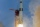In the arithmetic sequence a1 = 4.8, d = 0.4. How many consecutive members, starting with the first, need to be added so that the sum is greater than 170?
• When 5When 5 is subtracted from both my numerator and denominator I become 3/4. What fraction i am?
• Petra's testThe answer will be given 8 marks, and the wrong answer will be deducted 3 marks. The total number of marks obtained by the school is 36. If Petra answered six questions correctly,   how many questions were answered incorrectly by the student from the scho
• Row for lunchThere are less than 66 people in a row for lunch. In front of Eva, there are 28 students. Behind Martin, there are 30 people. Between Eva and Martin, there are 17 people. How many students stand behind Eva if Martin stands in front of Eva?
• Tower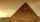Charles built a tower of cubes with an edge 2 cm long. In the lowest layer there were 6 cubes (in one row) in six rows, in each subsequent layer always 1 cube and one row less. What volume in cm3 did the whole tower have?
• Two diceWe roll two dice. What is the probability that the sum of the falling numbers is greater than 3?
• Squirrels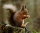The squirrels discovered a bush with hazelnuts. The first squirrel plucked one nut, the second squirrel two nuts, the third squirrel three nuts. Each new squirrel always tore one nut more than the previous squirrel. When they plucked all the nuts from the
• Ground levelThe temperature at ground level in Helsinki is 20°C lower than in London. The temperature in a basement flat in Helsinki is 5°C below the temperature at ground level. If it is -29°c in the basement, what is the temperature in London?
• GranddaughterIn 2014, the sum of the ages of Meghan's aunt, her daughter and her granddaughter was equal to 100 years. In what year was the granddaughter born, if we know that the age of each can be expressed as the power of two?
• Eight chairsThere are eight chairs by the table and one more child sitting on each one. There are two times more girls than boys. How many girls and how many boys can there be?
• On the floor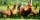You enter the room and there are 2 dogs, 4 horses, 1 giraffe and one duck on the bed, 3 chickens are flying over the chair. How many feet are on the floor?
• Quiz or testI have a quiz with 20 questions. Each question has four multiple-choice answers, A, B, C, D. THERE IS NO WAY TO KNOW THE CORRECT ANSWER OF ANY GIVEN QUESTION, but the answers are static, in that if the "correct" answer to #1 = C, then it will always be eq
• Sum of the seventeen numbersThe sum of the 17 different natural numbers is 154. Determine the sum of the two largest ones.
• Beet campaign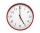The beet's field is being harvested. Beets are transported to 3 plants, 3 trucks. The first car returns to the field 3 times an hour, the second car after 30 minutes, and the third car 5 times an hour. All cars left at 8 in the morning. How many cars do w
• 45th birthdayThis year Mrs. Clever celebrated her 45th birthday. Her three children are now 7, 11 and 15 years old. In how many years will Mrs. Clever's age be equal to the sum of her children's years?
• The ticketsThe tickets to the show cost some integer number greater than 1. Also, the sum of the price of the children's and adult tickets, as well as their product, was the power of the prime number. Find all possible ticket prices.
• Pine's forest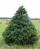There were so many pines in the forest that they were sequentially numbered 1, 2, 3,..., would use three times more digits than the pine trees alone. How many pine trees were there in the forest?
• Sum and ratioThe sum and ratio of two numbers is equal to 10. Which numbers are they?
• Self-counting machineThe self-counting machine works exactly like a calculator. The innkeeper wanted to add several three-digit natural numbers on his own. On the first attempt, he got the result in 2224. To check, he added these numbers again and he got 2198. Therefore, he a
• Find the sumFind the sum of all natural numbers from 1 and 100, which are divisible by 2 or 5

Do you have an exciting math question or word problem that you can't solve? Ask a question or post a math problem, and we can try to solve it.

We will send a solution to your e-mail address. Solved examples are also published here. Please enter the e-mail correctly and check whether you don't have a full mailbox.

Please do not submit problems from current active competitions such as Mathematical Olympiad, correspondence seminars etc...

Reason - math problems. Addition Problems.